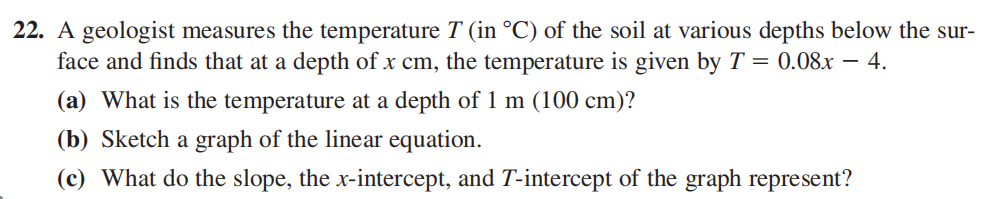### ¿Todavía tienes preguntas de matemáticas?

Pregunte a nuestros tutores expertos
Algebra
Pregunta22. A geologist measures the temperature $$T ($$ in $${ } ^ { \circ } C )$$ of the soil at various depths below the sur- face and finds that at a depth of $$x cm$$ , the temperature is given by $$T = 0.08 x - 4$$ .

(a) What is the temperature at a depth of $$1 m ( 100 cm )$$ ?

(b) Sketch a graph of the linear equation.

(c) What do the slope, the $$x$$ -intercept, and $$T$$ -intercept of the graph represent?

$$\left. \begin{array} { l } { \text { 22. (a) } 4 ^ { \circ } C \text { (b) } } \\ { \text { (c) The slope represents an increase of } 0.08 ^ { \circ } C \text { for each } } \\ { \text { one- centimeter increase in depth, the } x \text { - intercept is the depth } } \\ { \text { at which the temperature is } 0 ^ { \circ } C \text { , and the } T \text { - intercept is the } } \\ { \text { temperature at ground level. } } \end{array} \right.$$